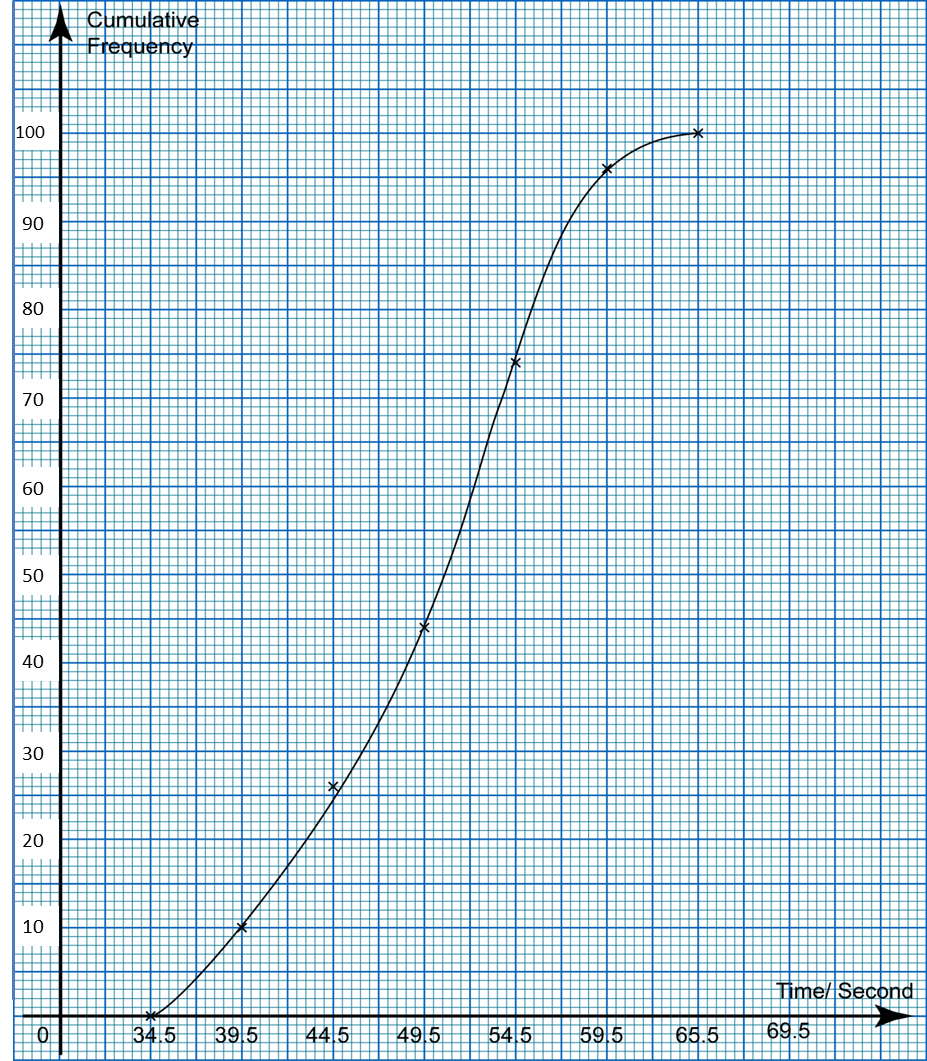# 7.6 Measures of Dispersion

7.6 Measures of Dispersion

(A) Determine the range of a set of data
1. For an ungrouped data,
Range = largest value – smallest value.

2.
For a grouped data,
Range = midpoint of the last class – midpoint of the first class.

Example 1:
Determine the range of the following data.
(a) 720, 840, 610, 980, 900

(b)
 Time (minutes) 1 – 6 7 – 12 13 – 18 19 – 24 25 – 30 Frequency 3 5 9 4 4

Solution:
(a)
Largest value of the data = 980
Smallest value of data = 610
Range = 980 – 610 = 370

(b)
Midpoint of the last class
= ½ (25 + 30) minutes
= 27.5 minute

Midpoint of the first class
= ½ (1 + 6) minutes
= 3.5 minute

Range = (27.5 – 3.5) minute = 24 minutes

(B) Medians and Quartiles
1. The first quartile (Q1)is a number such that 1 4 of the total number of data that has a value less than the number.

2.
The median is the second quartile which is the value that lies at the centre of the data.

3. The third quartile (Q3) is a number such that 3 4of the total number of data that has a value less than the number.

4. The interquartile range is the difference between the third quartile and the first quartile.

 Interquartile range = third quartile – first quartile

Example 2:The ogive in the diagram shows the distribution of time (to the nearest second) taken by 100 students in a swimming competition. From the ogive, determine
(a) the median,
(b) the first quartile,
(c) the third quartile
(d) the interquartile range of the time taken.

Solution:$\begin{array}{l}\text{(a)}\frac{1}{2}\text{of 100 students}=\frac{1}{2}×100=50\\ \text{From the ogive, median,}M=50.5\text{second}\\ \\ \text{(b)}\frac{1}{4}\text{of 100 students}=\frac{1}{4}×100=25\\ \text{From the ogive, first quartile,}{Q}_{1}\text{=}44.5\text{second}\\ \\ \text{(c)}\frac{3}{4}\text{of 100 students}=\frac{3}{4}×100=75\\ \text{From the ogive, third quartile,}{Q}_{3}\text{= 5}4.5\text{second}\end{array}$

(d)
Interquartile range
= Third quartile – First quartile
= 54.5 – 44.5
= 10.0 second